Logical Operators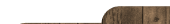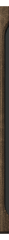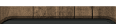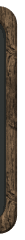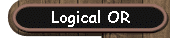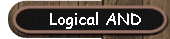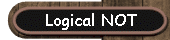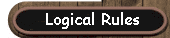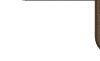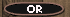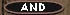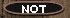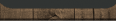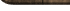Run your mouse over the panel at the left to see information about the logical operators.

A single comparison in an if statement often is not enough to determine whether data matches your criteria.  For example, you may need to check to see if a user enters a number within a certain range.  To accomplish this task, you will need the logical operators in your if statement.

These logical operators which result in a TRUE/FALSE condition are also referred to as Boolean Operators.

 It's time to remember those truth tables from Mathematics!  Yeek!!

 && (AND) (3 + 2 = 5) && (6 + 2 = 8)   TRUE (4 + 3 = 9) && (3 + 3 = 6)   FALSE | | (OR)   found above the backslash on the keyboard (3 + 6 = 2) || (4 + 1 = 5)      TRUE (1 + 1 = 3) || (3 + 3 = 9)      FALSE ! (NOT) !(4 + 3 = 5)      TRUE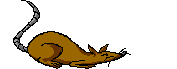BEWARE: Math:             2 < x < 10    OK Computer:    if (2 < x < 10)   NO!!!      The computer will only check the first condition 2 < x.               if ((2 < x) && (x < 10))  YES

 Remember DeMorgan's Laws: Mathematical Version: Programming Version: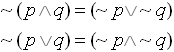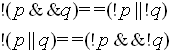Equivalent statements: if (!(num >= 0 && num <= 65)) if (num < 0 || num > 65)